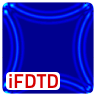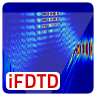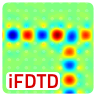# Educational Tools

Tutorials to get to know The Interactive FDTD Toolbox. Please feel free to give us some feedback if you like.

## Perfectly Matched Layers (PML) - iFDTD TutorialIn this first tutorial we want to demonstrate the effects of perfectly matched layer boundary conditions and get to know the interactive FDTD toolbox.

## Total Internal Reflection in a Prism - iFDTD TutorialIn this second tutorial we will show how to define a refractive index profile $$n\left(\mathbf{r}\right)$$ within the interactive FDTD toolbox. We will simulate the total internal reflection in a glass prism using a Gaussian shaped beam.

## Light Propagation in a Photonic Crystal - iFDTD TutorialIn the third tutorial of the interactive FDTD toolbox we will simulate the light propagation inside a photonic crystal. We will see that it will be very intuitive to understand the reflection at a defect and the use of a photonic crystal as beam splitter.